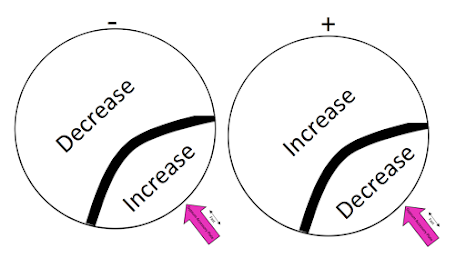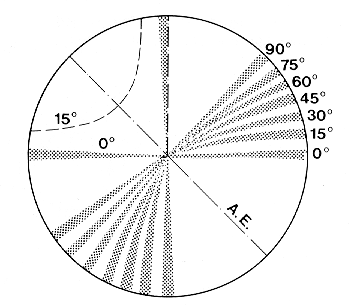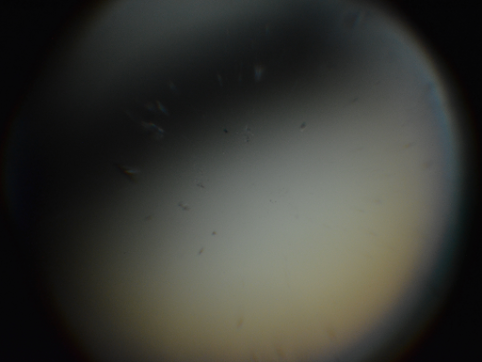Practice Quiz 6
For this exercise, please watch the first tutorial video before answering the questions. You are encouraged to use the video as much as you would like to answer the following questions. You will have an opportunity at the end to check your answers and take the quiz again for practice.
For a given mineral, which grain would you choose when looking for a biaxial optic axis centered interference figure?
In the video below, which mineral would be the best choice to use to obtain a biaxial optic axis centered interference figure?
The labels in the video are directly on the grain.
Use this diagram for the next two questions:In the video below the isogyre is off centered but we can still figure out the optic sign. Is the example biaxial positive or biaxial negative?
In the video below the isogyre is off centered but we can still figure out the optic sign. Is the example biaxial positive or biaxial negative?
In the picture below, what is the 2V angle?